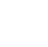Back to Chapter
Java IntroductionJava BasicsBranching and LoopingClasses, Objects, and MethodsArrays, Strings, and VectorsPackagesErrors and ExceptionApplet ProgrammingGraphics ProgrammingManaging I/O FilesJava Database Connectivity# Java Graphics class

To perform graphics like shapes, lines, textbox, label, checkbox, frames, and menu bars in java, the Graphics class is used. The Graphics class defines a number of drawing functions, Each shape can be drawn edge-only or filled. To draw shapes on the screen, we may call one of the methods available in the Graphics class. The most commonly used drawing methods included in the graphics class are listed below.1. Lines:

The simplest shape we can draw with the graphics class is a line. The drawLine() method takes two pairs of coordinates, (x1, y1) and (x2, y2) as arguments and draws a line between them. The g is the Graphics object passed to the paint() method.

syntax:

void drawLine(int startX, int startY, int endX, int endY);

Example:

// Lines.java
//Drawing Lines
import java.awt.*;
import java.applet.*;

public class Lines extends Applet
{
public void paint(Graphics g)
{
g.drawLine(0,0,100,100);
g.drawLine(0,100,100,0);
g.drawLine(40,25,250,180);
g.drawLine(5,290,80,19);
}
}

//Lines.html
<html>
<applet code=Lines.class
width=300 Height=250>
</applet>
</html>

output:

# 2. Rectangle:

The drawRect() and fillRect() methods display an outlined and filled rectangle, respectively. The upper-left corner of the rectangle is at(top, left). The dimensions of the rectangle are specified by width and height. Use drawRoundRect() or fillRoundRect() to draw a rounded rectangle. A rounded rectangle has rounded corners.

syntax:

void drawRect(int top, int left, int width, int height);
void fillRect(int top, int left, int width, int height);
void drawRoundRect(int top,int left,int width, int height int Xdiameter, int YDiameter);
void fillRoundRect(int top,int left,int width, int height int Xdiameter, int YDiameter);

Example:

//Rectangle.java
import java.awt.*;
import java.applet.*;

public class Rectangle extends Applet
{
public void paint(Graphics g)
{
g.drawRect(10,10,60,50);
g.fillRect(100,100,100,0);
g.drawRoundRect(190,10,60,50,15,15);
g.fillRoundRect(70,90,140,100,30,40);
}
}

//Rectangle.html
<html>
<applet code=Rectangle.class
width=300 Height=250>
</applet>
</html>

output:

# 3. Circles and Ellipses:

The Graphics class does not contain any method for circles or ellipses. To draw an ellipse, use drawOval(). To fill an ellipse, use fillOval(). To draw a circle, specify a square as the bounding rectangle i.e get height = width. The ellipse is drawn within a bounding rectangle whose upper-left corner is specified by (top, left) and whose width and height are specified by width and height.

syntax:

void drawOval(int top, int left, int width, int height);
void fillOval(int top, int left, int width, int height);

Example:

//Ellipses.java
import java.awt.*;
import java.applet.*;

public class Ellipses extends Applet
{
public void paint(Graphics g)
{
g.drawOval(10,10,60,50);
g.fillOval(100,10,75,50);
g.drawOval(190,10,90,30);
g.fillOval(70,90,140,100);
}
}
//Ellipses.html
<html>
<applet code=Ellipses.class
width=300 Height=250>
</applet>
</html>

output:

# 4. Drawing Arcs:

An arc is a part of the oval. Arcs can be drawn with draw Arc() and fillArc() methods. The arc is bounded by the rectangle whose upper-left corner is specified by (top, left) and whose width and height are specified by width and height. The arc is drawn from the start Angle through the angular distance specified by the sweep Angle. Angles are specified in degree. The arc is drawn counterclockwise if sweep Angle is positive, and clockwise if sweet Angle is negative.

syntax:

void drawArc(int top, int left, int width, int height, int startAngle, int sweetAngle);
void fillArc(int top, int left, int width, int height, int startAngle, int sweetAngle);

Example:

//Arcs.java
import java.awt.*;
import java.applet.*;

public class Arcs extends Applet
{
public void paint(Graphics g)
{
g.drawArc(10,40,70,70,0,75);
g.fillArc(100,40,70,70,0,75);
g.drawArc(10,100,70,80,0,175);
g.fillArc(100,100,70,90,0,270);
g.drawArc(200,80,80,80,0,180);
}
}
//Arcs.html
<html>
<applet code=Arcs.class
width=300 Height=250>
</applet>
</html>

output:

# 5. Polygons:

Polygons are shapes with many sides. It may be considered a set of lines connected. Use drawPolygon() and fillPolygon() to draw arbitrarily shaped figures. The polygon's endpoints are specified by the coordinate pairs contained within the x and y arrays. The number of points defined by x and y is specified by numPoints. Obviously, x and y arrays should be of the same size and we must repeat the first point at the end of the array to close the polygon.

syntax:

void drawPolygon(int ,[] , int y[], int numPointer);
void fillPolygon(int ,[] , int y[], int numPointer);

Example:

//Polygon.java
import java.awt.*;
import java.applet.*;

public class Polygon extends Applet
{
public void paint(Graphics g)
{
int xpoints[]={30,200,30,200,30};
int ypoints[]={30,30,200,200,30};
int num=5;
g.drawPolygon(xpoints,ypoints,num);
}
}
//Polygon.html
<html>
<applet code=Polygon.class
width=300 Height=250>
</applet>